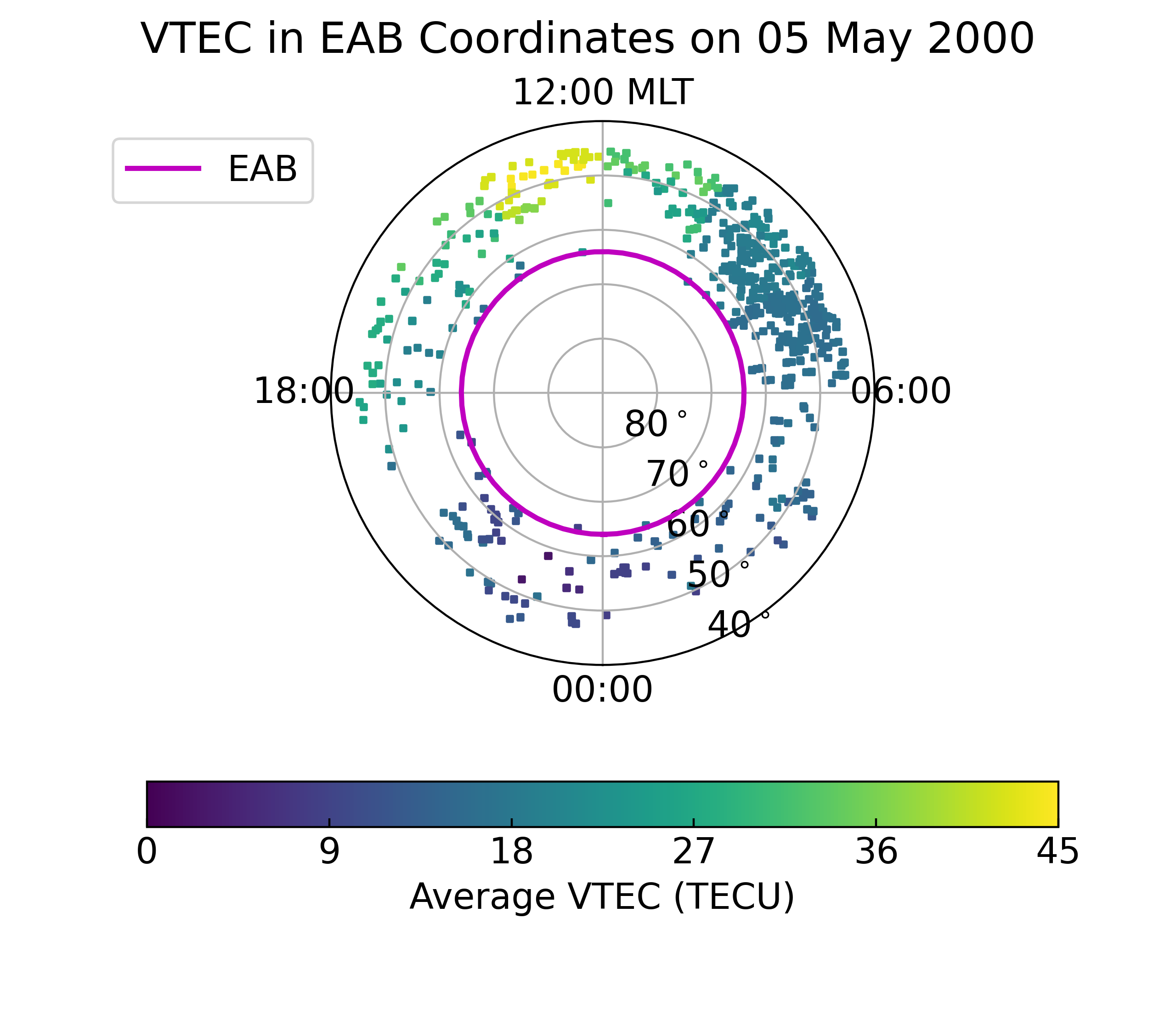# Using data from pysat (with EAB)¶

This example showcases Equatorward Auroral Boundaries (EABs), while demonstrating how to use `pysat` with `ocbpy`.

## Using Equatorward Auroral Boundaries¶

EABs may be loaded using the `EABoundary` class. This is a wrapper for the `OCBoundary` class with different defaults more appropriate for EABs. Currently, IMAGE and DMSP SSJ both have EAB data. This example uses the default file for IMAGE from the Northern hemisphere. It is very similar to Getting Started, the first example in this section.

```import ocbpy

eab = ocbpy.EABoundary(instrument='image', hemisphere=1)
print(eab)

EABoundary file: ~/ocbpy/ocbpy/boundaries/image_north_circle.eab
Source instrument: IMAGE
Boundary reference latitude: 64.0 degrees

305861 records from 2000-05-03 02:41:43 to 2002-10-31 20:05:16

YYYY-MM-DD HH:MM:SS Phi_Centre R_Centre R
------------------------------------------
2000-05-03 02:41:43 251.57 5.45 14.16
2000-05-05 11:35:27 111.80 2.34 25.12
2002-10-31 20:03:16 354.41 6.22 20.87
2002-10-31 20:05:16 2.87 14.67 13.10

Uses scaling function(s):
ocbpy.ocb_correction.circular(**{})
```

To prepare to use the EAB data, find the time with the first quality boundary. The expected record index, `eab.rec_ind`, is `1`.

```eab.get_next_good_ocb_ind()
```

Total Electron Content (TEC) is one of the most valuable ionospheric data sets. Madrigal provides Vertical TEC (VTEC) maps from the 1990’s onward that specify the median VTEC in 1 degree x 1 degree geographic latitude x longitude bins. The pysat package, pysatMadrigal has an instrument for obtaining, managing, and processing this VTEC data. To run this example, you must have `pysatMadrigal` installed. After setting up `pysat`, download the file needed for this example using the following commands.

```import pysat

# Replace `user` with a string holding your name and `password` with your
# email.  Madrigal uses these to demonstrate their utility to funders.
print(tec.files.files)

2000-05-04    gps000504g.001.netCDF4
2000-05-05    gps000505g.001.netCDF4
2000-05-06    gps000506g.001.netCDF4
dtype: object
```

`pysat` makes it possible to perform well-defined data analysis prodedures while loading the desired data. The `ocbpy.instrument.pysat_instrument` module contains functions that may be applied using the `pysat` custom interface. However, before this can be done the magnetic locations need to be calculated. This can be done by writing an appropriate function that takes the `pysat.Instrument` object as input and updates it within the function.

```import aacgmv2
import numpy as np

Parameters
----------
inst : pysat.Instrument
Data object
lat : str
Geodetic latitude key (default='gdlat')
lon : str
Geographic longitude key (default='glon')
alt : str
Geodetic altitude key (default='gdalt')
"""
# Initalize the data arrays
mlat = np.full(shape=tuple(tec.data.dims[kk]
for kk in ['time', lat, lon]),
fill_value=np.nan)
mlt = np.full(shape=mlat.shape, fill_value=np.nan)

# Cycle through all times, calculating magnetic locations
for i, dtime in enumerate(inst.index):
for j, gdlat in enumerate(inst[lat].values):
height = inst[alt][i, j].values
if not np.isnan(height).all():
mlat[i, j], mlon, r = aacgmv2.convert_latlon_arr(
gdlat, inst[lon].values, height, dtime)
mlt[i, j] = aacgmv2.convert_mlt(mlon, dtime)

# Assign the magnetic data to the input Instrument
inst.data = inst.data.assign({"mlat": (("time", lat, lon), mlat),
"mlt": (("time", lat, lon), mlt)})
return
```

Assign this function and the ocbpy function in the desired order of operations.

```tec.custom_attach(add_mag_coords)
kwargs={'ocb': eab, 'mlat_name': 'mlat',
'mlt_name': 'mlt', 'max_sdiff': 150})
print(tec.variables)

['time', 'gdlat', 'glon', 'dtec', 'gdalt', 'tec', 'mlat', 'mlt', 'mlat_ocb',
'mlt_ocb', 'r_corr_ocb']
```

Now we have the EAB coordinates for each location in the Northern Hemisphere where a good EAB was found within 2.5 minutes of the data record. This time difference was chosen because the VTEC data has a 5 minute resolution.

Now, let’s plot the average of the VTEC poleward of the EAB. To do this we will first need to calculate these averages.

```del_lat = 2.0
del_mlt = 2.0
ave_lat = np.arange(eab.boundary_lat, 90, del_lat)
ave_mlt = np.arange(0, 24, del_mlt)
ave_tec = np.full(shape=tec['tec'].shape, fill_value=np.nan)

for lat in ave_lat:
for mlt in ave_mlt:
# We are not overlapping bins, so don't need to worry about MLT
# rollover from 0-24
sel_tec = tec['tec'].where(
(tec['mlat_ocb'] > lat) & (tec['mlat_ocb'] <= lat + del_lat)
& (tec['mlt_ocb'] >= mlt) & (tec['mlt_ocb'] < mlt + del_mlt))
inds = np.where(~np.isnan(sel_tec.values))
if len(inds) > 0:
ave_tec[inds] = np.nanmean(sel_tec.values)
```

Now let us plot these averages at the EAB location of each measurement. This will provide us with knowledge of the coverage as well as knowledge of the average behaviour.

```# Initialise the figure
fig = plt.figure()
fig.suptitle("VTEC in EAB Coordinates on {:}".format(
tec.date.strftime('%d %B %Y')))
ax.set_theta_zero_location("S")
ax.xaxis.set_ticks([0, 0.5 * np.pi, np.pi, 1.5 * np.pi])
ax.xaxis.set_ticklabels(["00:00", "06:00", "12:00 MLT", "18:00"])
ax.set_rlim(0, 40)
ax.set_rticks([10, 20, 30, 40])
ax.yaxis.set_ticklabels(["80\$^\circ\$", "70\$^\circ\$", "60\$^\circ\$",
"50\$^\circ\$"])

lon = np.arange(0.0, 2.0 * np.pi + 0.1, 0.1)
lat = np.ones(shape=lon.shape) * (90.0 - eab.boundary_lat)
ax.plot(lon, lat, "m-", linewidth=2, label="EAB")

# Plot the VTEC data
tec_lon = tec['mlt_ocb'].values * np.pi / 12.0
tec_lat = 90.0 - tec['mlat_ocb'].values
tec_max = np.ceil(np.nanmax(ave_tec))
con = ax.scatter(tec_lon, tec_lat, c=ave_tec, marker="s",
cmap=mpl.cm.get_cmap("viridis"), s=5, vmin=0, vmax=tec_max)

# Add a colourbar and labels
tticks = np.linspace(0, tec_max, 6, endpoint=True)
cb = fig.colorbar(ax.collections, ax=ax, ticks=tticks,
orientation='horizontal')
cb.set_label('Average VTEC (TECU)')
ax.legend(fontsize='medium', bbox_to_anchor=(0.0, 1.0))
```